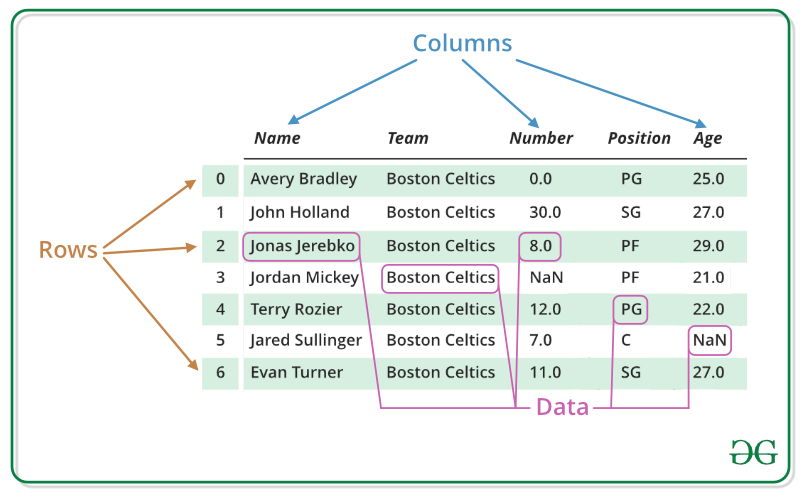Related Articles
R – Data Frames
• Last Updated : 30 Jun, 2020

R is an open-source programming language that is widely used as a statistical software and data analysis tool. DataFrames in R are generic data objects of R which are used to store the tabular data. Data frames can also be interpreted as mattresses where each column of a matrix can be of the different data types. DataFrame is made up of three principal components, the data, rows, and columns.#### Create Dataframe

To create a data frame in R use `data.frame()` command and then pass each of the vectors you have created as arguments to the function.
Example:

 `# R program to create dataframe`` ` `# creating a data frame``friend.data <``-` `data.frame(``    ``friend_id ``=` `c(``1``:``5``), ``    ``friend_name ``=` `c(``"Sachin"``, ``"Sourav"``, ``                    ``"Dravid"``, ``"Sehwag"``, ``                    ``"Dhoni"``),``    ``stringsAsFactors ``=` `FALSE``)``# print the data frame``print``(friend.data)`

Output:

```      friend_id friend_name
1         1      Sachin
2         2      Sourav
3         3      Dravid
4         4      Sehwag
5         5       Dhoni
```

#### Get the Structure of the Data Frame

One can get the structure of the data frame using `str()` function in R. It can display even the internal structure of large lists which are nested. It provides one liner output for the basic R objects letting the user know about the object and its constituents.
Example:

 `# R program to get the``# structure of the data frame`` ` `# creating a data frame``friend.data <``-` `data.frame(``    ``friend_id ``=` `c(``1``:``5``), ``    ``friend_name ``=` `c(``"Sachin"``, ``"Sourav"``, ``                    ``"Dravid"``, ``"Sehwag"``, ``                    ``"Dhoni"``),``    ``stringsAsFactors ``=` `FALSE``)``# using str()``print``(``str``(friend.data))`

Output:

```'data.frame':    5 obs. of  2 variables:
\$ friend_id  : int  1 2 3 4 5
\$ friend_name: chr  "Sachin" "Sourav" "Dravid" "Sehwag" ...
NULL
```

#### Summary of data in the data frame

In R data frame, the statistical summary and nature of the data can be obtained by applying `summary()` function. It is a generic function used to produce result summaries of the results of various model fitting functions. The function invokes particular methods which depend on the class of the first argument.
Example:

 `# R program to get the``# summary of the data frame`` ` `# creating a data frame``friend.data <``-` `data.frame(``    ``friend_id ``=` `c(``1``:``5``), ``    ``friend_name ``=` `c(``"Sachin"``, ``"Sourav"``, ``                    ``"Dravid"``, ``"Sehwag"``, ``                    ``"Dhoni"``),``    ``stringsAsFactors ``=` `FALSE``)``# using summary()``print``(summary(friend.data)) `

Output:

```    friend_id friend_name
Min.   :1   Length:5
1st Qu.:2   Class :character
Median :3   Mode  :character
Mean   :3
3rd Qu.:4
Max.   :5
```

#### Extract Data from Data Frame

Extract data from a data frame means that to access its rows or columns. One can extract specific column from a data frame using it’s column name.
Example:

 `# R program to extract``# data from the data frame`` ` `# creating a data frame``friend.data <``-` `data.frame(``    ``friend_id ``=` `c(``1``:``5``), ``    ``friend_name ``=` `c(``"Sachin"``, ``"Sourav"``, ``                    ``"Dravid"``, ``"Sehwag"``, ``                    ``"Dhoni"``),``    ``stringsAsFactors ``=` `FALSE``)`` ` `# Extracting friend_name column``result <``-` `data.frame(friend.data\$friend_name)``print``(result)`

Output:

```    friend.data.friend_name
1                  Sachin
2                  Sourav
3                  Dravid
4                  Sehwag
5                   Dhoni
```

#### Expand Data Frame

A data frame in R can be expanded by adding new columns and rows to the already existing data frame.
Example:

 `# R program to expand``# the data frame`` ` `# creating a data frame``friend.data <``-` `data.frame(``    ``friend_id ``=` `c(``1``:``5``), ``    ``friend_name ``=` `c(``"Sachin"``, ``"Sourav"``, ``                    ``"Dravid"``, ``"Sehwag"``, ``                    ``"Dhoni"``),``    ``stringsAsFactors ``=` `FALSE``)`` ` `# Expanding data frame``friend.data\$location <``-` `c(``"Kolkata"``, ``"Delhi"``, ``                       ``"Bangalore"``, ``"Hyderabad"``,``                       ``"Chennai"``)``resultant <``-` `friend.data``# print the modified data frame``print``(resultant)`

Output:

```    friend_id friend_name  location
1         1      Sachin   Kolkata
2         2      Sourav     Delhi
3         3      Dravid Bangalore
4         4      Sehwag Hyderabad
5         5       Dhoni   Chennai
```

In R, one can perform various types of operation on a data frame like accessing rows and columns, selecting the subset of the data frame, editing data frames, delete rows and columns in a data frame, etc. Please refer to DataFrame Operations in R to know about all types of operations that can be performed on a data frame.

Attention reader! Don’t stop learning now. Get hold of all the important DSA concepts with the DSA Self Paced Course at a student-friendly price and become industry ready.

My Personal Notes arrow_drop_up